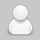# 【FT12短网址】借助JavaScript实现几种常见的排序算法

www.ft12.com6年前 (2017-08-15)短网址资讯1693

• 冒泡排序

• 选择排序

• 插入排序

• 合并排序

• 快速排序

1.冒泡排序

1.依次比较相邻的两个数，如果第一个比第二个小，不变。如果第一个比第二个大，调换顺序。一轮下来，最后一个是最大的数

2.对除了最后一个之外的数重复第一步，直到只剩一个数

`function bubbleSort(myArray){  var len = myArray.length;  var i;  var j;  var stop;  for (i = 0; i < len - 1; i++){    for (j = 0, stop = len - 1 - i; j < stop; j++){      if (myArray[j] > myArray[j + 1]){        swap(myArray, j, j + 1);      }    }  }  return myArray;}function swap(myArray, p1, p2){  var temp = myArray[p1];  myArray[p1] = myArray[p2];  myArray[p2] = temp;}`

2.选择排序

1.找出最小的数，和第一个交换位置

2.在剩下的数中，找出最二小的数，放在第二个

3.依次类推，排出顺序

`function selectionSort(myArray){    var len = myArray.length,        min;    for (i=0; i < len; i++){        // 将当前位置设为最小值        min = i;        // 检查数组其余部分是否更小        for (j=i+1; j < len; j++){            if (myArray[j] < myArray[min]){                min = j;            }        }        // 如果当前位置不是最小值，将其换为最小值        if (i != min){            swap(myArray, i, min);        }    }    return myArray;}function swap(myArray, p1, p2){    var temp = myArray[p1];    myArray[p1] = myArray[p2];    myArray[p2] = temp;}`

3.插入排序

1.把数组分为[已排序]和[未排序]两部分,第一个数为[已排序]，其余为[未排序]

2.从[未排序]抽出第一个数，和[已排序]部分比较，插入到合适的位置

`function insertionSort(myArray) {    var len     = myArray.length,     // 数组的长度        value,                      // 当前比较的值        i,                          // 未排序部分的当前位置        j;                          // 已排序部分的当前位置    for (i=0; i < len; i++) {        // 储存当前位置的值        value = myArray[i];        /*         * 当已排序部分的当前元素大于value，         * 就将当前元素向后移一位，再将前一位与value比较         */        for (j=i-1; j > -1 && myArray[j] > value; j--) {            myArray[j+1] = myArray[j];        }        myArray[j+1] = value;    }    return myArray;}`

4.合并排序(分而治之)

1.不断将数组对半分，直到每个数组只有一个

2.将分出来的部分重新合并

3.合并的时候按顺序排列

`// 被拆分的数组重新合并function merge(left, right) {    var result = [],        left_index = 0,        right_index = 0;    // 将两个数组合并    // 合并的时候按从小到大的顺序    while (left_index < left.length && right_index < right.length) {        if (left[left_index] < right[right_index]) {            result.push(left[left_index++]);        } else {            result.push(right[right_index++]);        }    }    // 和其他数组拼接    return result.concat(left.slice(left_index)).concat(right.slice(right_index));}function mergeSort(myArray) {    // 只有一个数的时候退出递归    if (myArray.length < 2) {        return myArray;    }    var middle = Math.floor(myArray.length / 2),        left = myArray.slice(0, middle),        right = myArray.slice(middle);    // 递归    // 不断拆分只到一个数组只有一个数    return merge(mergeSort(left), mergeSort(right));}`

5.快速排序

1.以一个数为基准(中间的数)，比基准小的放到左边，比基准大的放到右边

2.再按此方法对这两部分数据分别进行快速排序（递归进行）

3.不能再分后退出递归，并重新将数组合并

`var quickSort = function(myArray) {　　// 当被分的数组只剩一个时，退出递归if (myArray.length <= 1) {return myArray;   }// 中间基准值的indexvar pivotIndex = Math.floor(myArray.length / 2);　　// 基准值var pivot = myArray.splice(pivotIndex, 1);　　var left = [];　　var right = [];　　// 小的放左边，大的放右边for (var i = 0; i < myArray.length; i++) {　　　　if (myArray[i] < pivot) {　　left.push(myArray[i]);　       } else {right.push(myArray[i]);　       }　　   }　　// 递归// 把数组合并在一起return quickSort(left).concat([pivot], quickSort(right));};`

### 群殴百度之后，谁才能改写BAT的阵营?

6月26日，京东收盘价43.08元，总市值611.49亿美金，已很接近百度同期的总市值619.73亿美金，“JAT”在喧嚣中被提出。在BAT的阵营之中，不管是从营收和市值的角度，还是从业务发展的趋势，支付宝和微信都享有更大市场份额、更高增长...

### 巨头逐鹿消费金融，京东派快递员推销白条

[ FT12短网址 ] 最近一两年，在互联网金融公司掀起上市热潮的背景下，互联网消费信贷的发展在今年进入快车道，成了巨头们角逐的领域。先后出台了京东白天、芝麻花呗、腾讯借呗等等。图片来自网络互联网消费信贷的发展在今年进入快车道。近...

### FT12短网址：苏宁和国美同时抢滩汽车市场 车源或为成败要素

2017年7月15日，苏宁易购轿车超市（以下简称“轿车超市”）在南京中心商圈新街口开业，产品定位中高端，将O2O形式应用到轿车消费商场。苏宁方面表明，将来将在全国开设超越100家轿车超市。无独有偶，3月28日，国美电器控股在京举行轿车招商发...

### FT12短网址：绘制随机不规则三角彩条——小谈FT12短网址主页的实现

#### 发表评论◎欢迎参与讨论，请在这里发表您的看法和观点。